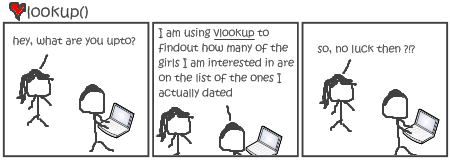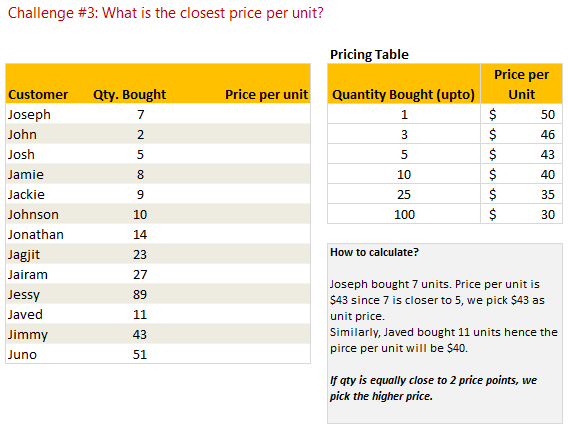# 3 Lookup Formula Challenges + 2 Jokes + 1 Link [VLOOKUP Week]

Share

“Start with a joke.” My boss used to say when I am nervous about an upcoming presentation. Although, I am not nervous to post this article, I think a joke will always help.

So here it goes:Now to more serious matters.VLOOKUP (and other lookup formulas) are very powerful and quite practical. They can fetch you the information you are looking for from a heap of data.

Now that we have seen the power of VLOOKUP thru several posts this week, I want to test your understanding of these formulas by presenting 3 challenges.

### Challenge # 1: Price After Discount

We come across this problem quite often. You have a list of discount codes and applicable quantity thresholds. For eg. you may sell an item at \$50, but if I buy more than 1 item, you will give a 10% discount. The discount goes up as I purchase more quantity.

Now, given a list of item quantities, how do you calculate the amount payable using lookup formulas? That is our first challenge.### Challenge # 2: Price after accumulated quantity discount

This is essentially same as above formula, but the discounts apply on accumulated quantities bought so far. For eg. I will get first item for 0% discount, 2nd and 3rd items for 10% discount, 4th item for 15% discount … 26th item for 50% discount etc.

Now, given a list of customer names and quantities they bought (in the same order), how do you calculate the amount payable for each transaction?### Challenge # 3: Closest price based on the quantity purchased

This is an interesting challenge. The price after discount is determined based on the quantity bought. For eg. the discount thresholds are 1, 3, 5, 10, 25 etc. Now, given a quantity of items bought, we determine the price by finding the closest threshold to it. So, a quantity of 7 will get the price from threshold 5 as against 10.### Few guidelines on solving these challenges:

Although the above problem might appear simple, the solution is not so straightforward.

• Use a variety of formulas: Do not just rely VLOOKUP. Instead experiment with formulas like SUMIF, COUNTIF, INDEX, MATCH etc. to get results
• Use helper columns: Break down the problem in to several steps and use helper columns to get the results
• Use pen & paper: Write down the logic first, then simulate it in excel using formulas. It clears your mind fast.
• Many solutions exist: Each problem can be solved in several different ways. So once you find a solution, feel free to explore other options
• Share your solutions: Use comments box to share your solutions with us. I am always looking for new ways to solve problems. So teach me…

### Solution to the Challenges:

Here is a workbook with one set of solutions  for the problems. As I said, many other solutions do exist. So use this workbook as an indication of what is possible.

### One Link to More VLOOKUP Awesomeness:

Debra at Contextures has chipped in with some interesting videos on VLOOKUP formulas. Check them out here.

### The 2nd Joke:

It is quite difficult to set an expectation and then meet it. More so with jokes. But do you know that Chandoo.org’s 404 pages show Excel error messages? For example go to http://chandoo.org/wp/missing_file/. Refresh the page to see a different message. 🙂

It is Diwali (the festival of lights) in India this weekend. So I am going to spend time with family, light some fireworks and relax. I wish you a happy Diwali if you celebrate one. Even otherwise, I wish a lot of light and warmth in to your life this year.### Get FREE Excel + Power BI Tips

Simple, fun and useful emails, once per week.

Learn & be awesome.

### Welcome to Chandoo.org

Thank you so much for visiting. My aim is to make you awesome in Excel & Power BI. I do this by sharing videos, tips, examples and downloads on this website. There are more than 1,000 pages with all things Excel, Power BI, Dashboards & VBA here. Go ahead and spend few minutes to be AWESOME.

Read my storyFREE Excel tips book

I will be presenting at two events in London in April 2020. Come & join me.

Chandoo is an awesome teacher
5/5

– Jason

Still on fence about Power BI? In this getting started guide, learn what is Power BI, how to get it and how to create your first report from scratch.

### 29 Responses to “3 Lookup Formula Challenges + 2 Jokes + 1 Link [VLOOKUP Week]”

1.Oli says:

If I have read the brief correctly then these are my solutions.
My solution to Challenge #1
=\$C7*(\$D\$5*(1-INDEX(\$F\$7:\$G\$12,MATCH(SUMPRODUCT(MAX((\$C7>=\$F\$7:\$F\$12)*(\$F\$7:\$F\$12))),\$F\$7:\$F\$12,0),2)))
My solution to Challenge #2
=\$C26*\$D\$24*(1-INDEX(\$F\$26:\$G\$31,MATCH(SUMPRODUCT(MAX(((SUMPRODUCT((INDEX(\$B\$26:\$B26,,)=\$B26)*\$C\$26:\$C26))>=\$F\$26:\$F\$31)*(\$F\$26:\$F\$31))),\$F\$26:\$F\$31,0),2))
BTW there is an Error in example Josh only gets 20% discount on second purchase
My solution to Challenge #3
=INDEX(\$F\$46:\$G\$51,MATCH(\$C46,\$F\$46:\$F\$51),2)

•vinicius says:

hi, im not that advanced hehe
whats the function of the "1" in the (1-index[...] formula?

•Sushil Kumar says:

This is wrong because your value of 26 unit after deduction Discount Amount is shown 975 ...

Actual value show is 650 after deduction so your formula is wrong

2.DQKennard says:

We should all have more festivals/holidays/celebrations that involve fireworks.

3.Ben says:

All of these answers require that you resort the discount table for quantity bought in descending order.

1)=(1-INDEX(\$G\$7:\$G\$12,MATCH(\$C7,\$F\$7:\$F\$12,-1)))*\$D\$5*\$C7

2) Solution to #2 is an array formula, so must hit ctrl+shft+enter.
{=((1-INDEX(\$G\$26:\$G\$31,MATCH(\$C26,\$F\$26:\$F\$31,-1)))*\$D\$24*\$C26)+IFERROR(MAX((\$B25:\$B\$26=\$B26)*(\$D25:\$D\$26)),0)}

3)=INDEX(\$G\$46:\$G\$51,MATCH(IF((INDEX(\$F\$46:\$F\$51,MATCH(\$C46,\$F\$46:\$F\$51,-1))-\$C46)<=(INDEX(\$F\$46:\$F\$51,MATCH(\$C46,\$F\$46:\$F\$51,-1))-INDEX(\$F\$46:\$F\$51,MATCH(\$C46,\$F\$46:\$F\$51,-1)+1))/2,INDEX(\$F\$46:\$F\$51,MATCH(\$C46,\$F\$46:\$F\$51,-1)),INDEX(\$F\$46:\$F\$51,MATCH(\$C46,\$F\$46:\$F\$51,-1)+1)),\$F\$46:\$F\$51,0))

4.Luke M says:

1) This is an array formula:
=C7*\$D\$5*(1-INDEX(\$G\$7:\$G\$12,MATCH(TRUE,C7<=\$F\$7:\$F\$12,0)))
2) Another array formula, similar to first:
=C26*\$D\$24*(1-INDEX(\$G\$26:\$G\$31,MATCH(TRUE,SUMIF(B\$26:B26,B26,C\$26:C26)<=\$F\$26:\$F\$31,0)))
3) Had to subtract 0.1 so lookup would pick larger price:
=LOOKUP(C46-0.1,(\$F\$46:\$F\$51+\$F\$45:\$F\$50)/2,\$G\$46:\$G\$51)

5.Elias says:

@Luke, your third formula fails when quantity is equal to 2

1) =(1-MIN(IF(\$F\$7:\$F\$12>=C7,\$G\$7:\$G\$12,0.5)))*\$D\$5*C7
Array Enter
2)=(1-MIN(IF(\$F\$26:\$F\$31>=C26+SUMIF(\$B\$25:B25,B26,\$C\$25:C25),\$G\$26:\$G\$31,0.5)))*\$D\$5*C26
Array Enter
3)=INDEX(\$G\$46:\$G\$51,MATCH(MIN(ABS(\$F\$46:\$F\$51-C46)),ABS(\$F\$46:\$F\$51-C46),0))
Array Enter

Regards

6.Luke M says:

@Elias
No it doesn't... Chandoo stated that in the case of qty being equally close to two data points, pick the higher price. The answer should be \$50. (this is what displays on my computer) What number are you getting?

7.Luke M says:

@Elias & Myself
Bah, I see now that the workbook (which I can't download) would have text in F45. Compensating by hard entering the data:
=LOOKUP(C47-0.1,(\$F\$46:\$F\$51+{0,1,3,5,10,25})/2,\$G\$46:\$G\$51)

8.Luke M says:

Correction again...
ARGH!!! I always mix up my commas and semi-colons...
=LOOKUP(C46-0.1,(\$F\$46:\$F\$51+{0;1;3;5;10;25})/2,\$G\$46:\$G\$51)

9.Elias says:

@Luke, have you checked the results of your latest formula?

Thanks

10.Luke M says:

@Elias
Yep, it's matching yours...is there another error being generated somewhere? I apologize, I don't have access to Chandoo's workbook, so I'm having to reconstruct

11.Elias says:

@Luke,

I replied before you changed the commas and semi-colons.

Regards

12.sixseven says:

These formulas require the discount table to be sorted in assending order, like they are in the example file:

Question 1: (paste in cell D7 and fill down)

=C7*\$D\$5*(1-INDEX(\$F\$7:\$G\$12,IF(C7=VLOOKUP(C7,\$F\$7:\$F\$12,1,TRUE),MATCH(VLOOKUP(C7,\$F\$7:\$F\$12,1,TRUE),\$F\$7:\$F\$12,0),MATCH(VLOOKUP(C7,\$F\$7:\$F\$12,1,TRUE),\$F\$7:\$F\$12,0)+1),2))

Question 2: (paste in cell D26 and fill down)

=C26*\$D\$24*(1-INDEX(\$F\$26:\$G\$31,IF(SUMIF(\$B\$26:B26,B26,\$C\$26:C26)=VLOOKUP(SUMIF(\$B\$26:B26,B26,\$C\$26:C26),\$F\$26:\$F\$31,1,TRUE),MATCH(VLOOKUP(SUMIF(\$B\$26:B26,B26,\$C\$26:C26),\$F\$26:\$F\$31,1,TRUE),\$F\$26:\$F\$31,0),MATCH(VLOOKUP(SUMIF(\$B\$26:B26,B26,\$C\$26:C26),\$F\$26:\$F\$31,1,TRUE),\$F\$26:\$F\$31,0)+1),2))

Question 3: (I had a little fun with this one. Use RANK() instead of the methods in formulas 1 & 2. Paste in cell D46 and fill down.)

=IF(INDEX(\$F\$46:\$F\$51,RANK(C46,(C46,\$F\$46:\$F\$51),1))-C46<=2,INDEX(\$F\$46:\$G\$51,RANK(C46,(C46,\$F\$46:\$F\$51),1),2),INDEX(\$F\$46:\$G\$51,RANK(C46,(C46,\$F\$46:\$F\$51),1)-1,2))

On a side note, these formulas were fun to determin the "up to" discount, but if I were creating this report for a customer, I would advise them to structure their discounts so that a new discount tier is achieved when a buying threshold is reached. I feel this would be better understood by the customer, and it would make your discounts looks more attractive from a sales perspective. The pricing table for the first scenario would look like this:

Qty = Discount
1 = 0%
2-3 = 10%
4-5 = 15%
6-10 = 20%
11-25 = 25%
26+ = 50%

13.sixseven says:

Just noticed that my formula #3 is broken for any Qty between 75 & 97.
|
This line is a test of a line break for future posts... 🙂

14.Tristan says:

Just saw that problem 3 was for price per unit, not total coast

revise to

=INDEX(\$G\$46:\$G\$51,MATCH(0,INDEX((\$F\$46:\$F\$51-C46)^2-MIN(INDEX((\$F\$46:\$F\$51-C46)^2,0,0)),0,0),0),1)

15.Unai says:

I have found a "solution" which gives different output than yours.

In #1: (1-VLOOKUP(C7,\$F\$7:\$G\$12,2,1))*C7*\$D\$5

This assumes that if you buy from 10 to 99 you get 20% discount, and if you buy 100 or more, 50%. I may have misunderstood the question.

In #2: (1-VLOOKUP(SUMPRODUCT((B26=\$B\$26:B26)*(\$C\$26:C26)),\$F\$26:\$G\$31,2,1))*C26*\$D\$24 and copy downwards the formula. Note that there are ranges that grow with rows.

Same assumption than in #1.

In #3: VLOOKUP(C46,\$F\$46:\$G\$51,2,1)

Here the formula is simple because the data are nicely sorted.

•Sushil Kumar says:

This is wrong because your value of 26 unit after deduction Discount Amount is shown 975 ...

Actual value show is 650 after deduction so your formula is wrong

16.Alejandro says:

Challenge #1:
=(C7*\$D\$5)-(IF(C7<=\$F\$7,\$G\$7,IF(C7<=\$F\$8,\$G\$8,IF(C7<=\$F\$9,\$G\$9,IF(C7<=\$F\$10,\$G\$10,IF(C7<=\$F\$11,\$G\$11,IF(C7<=\$F\$12,\$G\$12,"N/A"))))))*(C7*\$D\$5))

Challenge #2:
=(C26*\$D\$24)-(IF((SUMIF(\$B\$26:B26,B26,\$C\$26:C26))<=\$F\$26,\$G\$26,IF((SUMIF(\$B\$26:B26,B26,\$C\$26:C26))<=\$F\$27,\$G\$27,IF((SUMIF(\$B\$26:B26,B26,\$C\$26:C26))<=\$F\$28,\$G\$28,IF((SUMIF(\$B\$26:B26,B26,\$C\$26:C26))<=\$F\$29,\$G\$29,IF((SUMIF(\$B\$26:B26,B26,\$C\$26:C26))<=\$F\$30,\$G\$30,IF((SUMIF(\$B\$26:B26,B26,\$C\$26:C26))<=\$F\$31,\$G\$31,"N/A"))))))*(C26*\$D\$24))

Challenge #3
=VLOOKUP(C46,\$F\$46:\$G\$51,2,TRUE)

17.SS says:

Ch#1 - =\$D\$5*C7-INDEX(\$G\$6:\$G\$12,IFERROR(MATCH(C7,\$F\$6:\$F\$12,0), MATCH(C7,\$F\$6:\$F\$12,1)+1))*\$D\$5*C7
Ch#3 - =INDEX(\$G\$45:\$G\$51,MATCH(C46,\$F\$46:\$F\$51,1)+1)

Couldn't figure out #2!! 🙁

18.Drazen says:

It was an interesting challenge...
```1.) =IF(C7=INDEX(\$F\$7:\$G\$12; MATCH(C7;\$F\$7:\$F\$12;1);1); 1-INDEX(\$F\$7:\$G\$12;MATCH(C7;\$F\$7:\$F\$12;1);2); 1-INDEX(\$F\$7:\$G\$12;MATCH(C7;\$F\$7:\$F\$12;1)+1;2))* C7*\$D\$5 2.) This one was a little bit tricky :-) =IF(SUMIF(\$B\$26:B26;B26;\$C\$26:C26)= INDEX(\$F\$26:\$G\$31; MATCH(C26;\$F\$26:\$F\$31;1);1); (1-INDEX(\$F\$26:\$G\$31; MATCH(SUMIF(\$B\$26:B26;B26;\$C\$26:C26); \$F\$26:\$F\$31;1);2)); (1-INDEX(\$F\$26:\$G\$31; MATCH(SUMIF(\$B\$26:B26;B26;\$C\$26:C26); \$F\$26:\$F\$31;1)+1;2)))*C26*\$D\$24 3.) =IF(C46<=MEDIAN(INDEX(\$F\$46:\$F\$51;MATCH(C46;\$F\$46:\$F\$51;1);1) ;INDEX(\$F\$46:\$F\$51; MATCH(C46;\$F\$46:\$F\$51;1)+1;1)); INDEX(\$F\$46:\$G\$51; MATCH(C46;\$F\$46:\$F\$51;1);2); INDEX(\$F\$46:\$G\$51;MATCH(C46;\$F\$46:\$F\$51;1)+1;2))```
According to my testing results are same as Chandoo's are.

19.dhananjay says:

hi,
actually i guess many of the formulas are not working for the 3rd challenge.. i mean qty is close to 2 price pts, it should pick the higher price.. its working fine if \$50 is the first value in the price column...but if i change it to \$ 40 it should pick the higher price which is \$46 any help??

•Tristan says:

@dhananjay

Most of the solutions require, either explicitly or implicitly, that the prices are in descending order and the quantity is in ascending order.
This formula should decouple the order:
=LARGE(INDEX((INDEX(ABS(\$F\$46:\$F\$51-C46),,)=MIN(INDEX(ABS(\$F\$46:\$F\$51-C46),,)))*\$G\$46:\$G\$51,,),1)

20.dhananjay says:

@tristan

thanku.... it worked.. it worked well even without the index functions by pressing ctrl shift enter..
=LARGE(((ABS(\$F\$46:\$F\$51-C46)=MIN(ABS(\$F\$46:\$F\$51-C46)))*\$G\$46:\$G\$51),1)

21.tim says:

Challenge #1 enter the following formula in cell D7 and auto fill to the rest
=C7*\$D\$5*(1-VLOOKUP(C7,\$F6\$:\$G\$12,2,TRUE))

Challenge #2. Discount table must be sorted in descending order first. Then enter the following formula in cell D26 and auto fill to the rest
=C26*\$D\$24*(1-INDEX(\$G\$26:\$G\$31,MATCH(SUMIF(\$B\$26:B26,B26,\$C\$26:C26),\$F\$26:\$F\$31,-1)))

Challenge #3 enter the following formula in cell D46 and auto fill to the rest
=VLOOKUP(C46,\$F\$46:\$G\$51,2,TRUE)

22.Junaid Azhar doga says:

=C7*\$D\$5-C7*\$D\$5*VLOOKUP(C7,\$F\$7:\$G\$12,2,TRUE)

•Sushil Kumar says:

This is wrong because your value of 26 unit after deduction Discount Amount is shown 975 ...

Actual value show is 650 after deduction so your formula is wrong

23.Sushil Kumar says:

=(C7*\$D\$5)-(C7*\$D\$5)*ArrayFormula(max((C7>\$F\$7:\$F\$12)*\$G\$8:\$G\$13))

24.Sushmita Bhar says:

solution 1:
{=(1-INDEX(\$H\$7:\$H\$12,MATCH(TRUE,data1>=C7,0)))*C7*\$D\$5}
Solution 2:
{=(1-INDEX(\$H\$26:\$H\$31,MATCH(TRUE,data2>=SUMIF(\$B\$26:B26,B26,\$C\$26:C26),0)))*C26*\$D\$24}
Solution 3:
{=VLOOKUP(INDEX(data,MATCH(MIN(ABS(data-C46)),ABS(data-C46),0)),\$G\$46:\$H\$51,2,FALSE)}

### Get FREE Excel & Power-BI Newsletter

One email per week with Excel and Power BI goodness. Join 100,000+ others and get it free.